# Analogy: Math and Cooking

Author: kalid
Go to Source

If we had a recipe for pie, there’s a few things we might expect. If we doubled the ingredients, we’d probably get double the pie. But if we took the ingredients and cooked them separately, we wouldn’t expect to put them together and get our pie back. You can’t bake the eggs, flour, and sugar in separate ovens and plop them together at the end.

In math, we can get misleading intuitions about what can (or can’t) be rearranged.

After learning addition, we’ve memorized facts like 2 + 4 = 6. But this might stray into the idea that “whenever I see 2 and 4, I can simplify to 6”.

Although 2 + 4 = 6, but “baked(2) + baked(4)” is not “baked(6)”. Baking unmixed ingredients in the exponential oven we get:

\$2^2 + 4^2 neq 6^2\$

We can only confidently say:

\$(2 + 4)^2 = 6^2\$

We combine the ingredients, then bake the result. Exponents, like baking an apple pie, modify the original ingredients so they can’t be easily combined later. While we might recognize the original 2 and 4, they aren’t directly available. Two baked pies can’t be smashed together to consolidate the filling.

This confusion gummed me up in calculus, when learning derivatives (the bad boy of baking).

In algebra, we internalize rules like: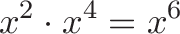$displaystyle{x^2 cdot x^4 = x^6}$

But our intuition leads us astray when we get to the derivative.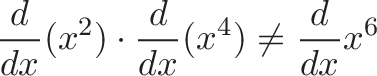$displaystyle{frac{d}{dx}(x^2) cdot frac{d}{dx}(x^4) neq frac{d}{dx}x^6}$

because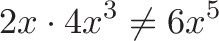$displaystyle{2x cdot 4x^3 neq 6x^5 }$

Raw polynomials can be multiplied, but the derivatives of multiplied polynomials can’t be rearranged so easily. Multiplication makes functions interact in a way that makes taking the derivative more complex: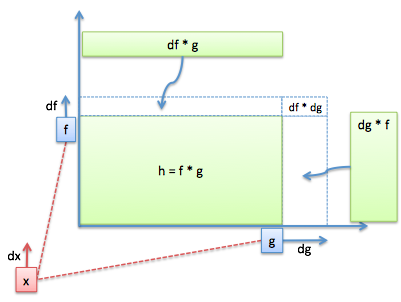Working through the Product Rule we get: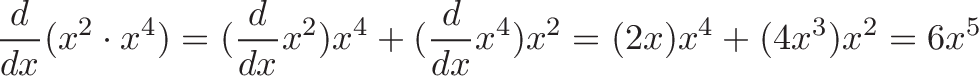$displaystyle{frac{d}{dx}(x^2 cdot x^4) = (frac{d}{dx}x^2)x^4 + (frac{d}{dx}x^4)x^2 = (2x)x^4 + (4x^3)x^2 = 6x^5}$

When learning Calculus, I was confused how standard interactions (like multiplication) needed special handling. I thought I was done learning new rules for “arithmetic”.

But no: functions, when multiplied, interact in funky ways. See how each side grows its own sliver of area (`df * g` and `dg * f`)? The functions being multiplied are “baked together” and the overall effect depends on them both, simultaneously. We can’t examine them in isolation (e.g., `df` or `dg` by itself).

Now, there are setups when the inputs can be processed separately and combined later (linear algebra). The cooking equivalent might be a smoothie: An apple/banana smoothie mixed with a peach/mango smoothie is the same as blending all ingredients in the beginning.

A common assumption is that operations are usually linear, but \$sin(a + b) not= sin(a) + sin(b)\$ and \$(a + b)^2 not= a^2 + b^2\$. Sorry, we have to carefully cook the ingredients if we want the math to taste right.

When our intuition for a math rule doesn’t make sense, ask “Are we making a pie, or a smoothie?”